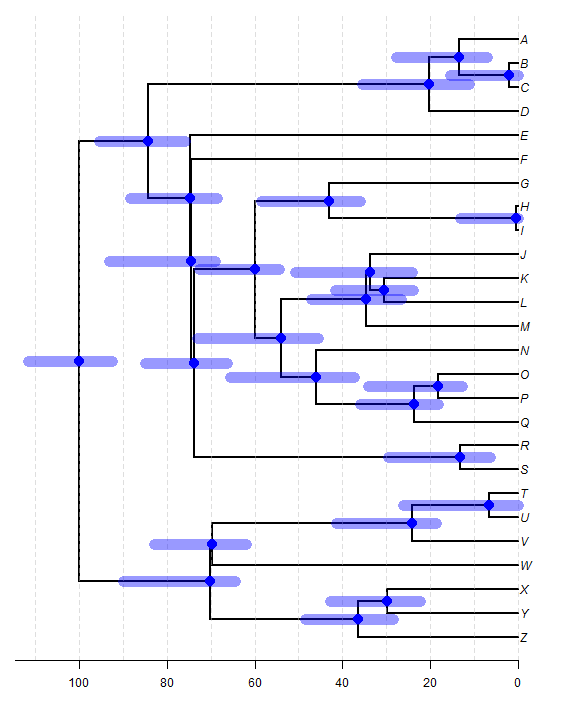## Wednesday, March 1, 2017

### Error bars on divergence times on a phylogeny plotted in R

Today a colleague at Universidad de los Andes (where I'm currently on sabbatical, BTW) asked me how to add error bars for divergence times to the nodes of a plotted phylogeny in R.

Well, as is the case with many things, I bet that there is a ton of different ways to do this; however, the following is one very simple technique.

In the following, I have a `"phylo"` object `tree`; and I also have a matrix (`CI`) containing the times before the present for the lower & upper confidence limits on each estimated divergence time corresponding to the nodes of my tree. Note that these CIs have been simulated to be intentionally assymetric - as such confidence intervals often are:

``````library(phytools)
## here are the data:
tree
``````
``````##
## Phylogenetic tree with 26 tips and 25 internal nodes.
##
## Tip labels:
##  Z, Y, X, W, V, U, ...
##
## Rooted; includes branch lengths.
``````
``````CI
``````
``````##    lower (MYBP) upper (MYBP)
## 27    111.80101    92.599420
## 28     90.13474    64.370663
## 29     48.45811    28.394226
## 30     42.77937    22.359999
## 31     83.01736    61.962733
## 32     41.48123    18.614973
## 33     26.10956     0.000000
## 34     95.60433    75.950823
## 35     88.41807    68.551308
## 36     93.32274    69.118385
## 37     84.96369    66.249093
## 38     29.45826     6.303606
## 39     72.61971    54.372320
## 40     73.15644    45.563312
## 41     65.70458    37.262456
## 42     35.86271    18.184414
## 43     34.17755    12.586219
## 44     47.11305    26.530968
## 45     50.74606    24.070106
## 46     41.64973    23.878326
## 47     58.47533    35.912961
## 48     13.19029     0.000000
## 49     35.42824    11.007959
## 50     27.73721     6.913533
## 51     15.31440     0.000000
``````

Now let's plot it:

``````plotTree(tree,xlim=c(110,-5),direction="leftwards",
mar=c(4.1,1.1,1.1,1.1),ftype="i")
abline(v=seq(0,120,by=10),lty="dashed",
col=make.transparent("grey",0.5))
axis(1,at=seq(0,120,by=20))
obj<-get("last_plot.phylo",envir=.PlotPhyloEnv)
for(i in 1:tree\$Nnode+Ntip(tree))
lines(x=c(CI[i-Ntip(tree),1],CI[i-Ntip(tree),2]),
y=rep(obj\$yy[i],2),lwd=11,lend=0,
col=make.transparent("blue",0.4))
points(obj\$xx[1:tree\$Nnode+Ntip(tree)],
obj\$yy[1:tree\$Nnode+Ntip(tree)],pch=19,col="blue",
cex=1.8)
``````(I added a few embellishments, but it should be straightforward to pick these out.)

I like it.

As with most such plots, it will tend to look better if expored as a PDF.

Note that because my CIs are in time for the present, I decide to plot my tree `"leftwards"`, but then flip my x axis to run from higher to lesser values using the argument value `xlim=c(110,-5)`.

Note also that in the example above I have assumed that the order of the rows of `CI` matches the order of the node indices in the tree.

These were simulated data, obviously. The code used to simulate these values was as follows:

``````tree<-pbtree(n=26,tip.label=LETTERS[26:1],scale=100)
h<-sapply(1:tree\$Nnode+Ntip(tree),nodeheight,tree=tree)
CI<-cbind(h-runif(n=length(h),min=10,max=20),h+runif(n=length(h),
min=5,max=10))
CI[CI>max(nodeHeights(tree))]<-max(nodeHeights(tree))
CI<-max(nodeHeights(tree))-CI
rownames(CI)<-1:tree\$Nnode+Ntip(tree)
colnames(CI)<-paste(c("lower","upper"),"(MYBP)")
``````

1.Dear Liam,

How can I get the matrix of CI from a posterior distribution of trees (empirical data)?
Thanks,
Aparna

2.I'm looking for the same

Note: due to the very large amount of spam, all comments are now automatically submitted for moderation.